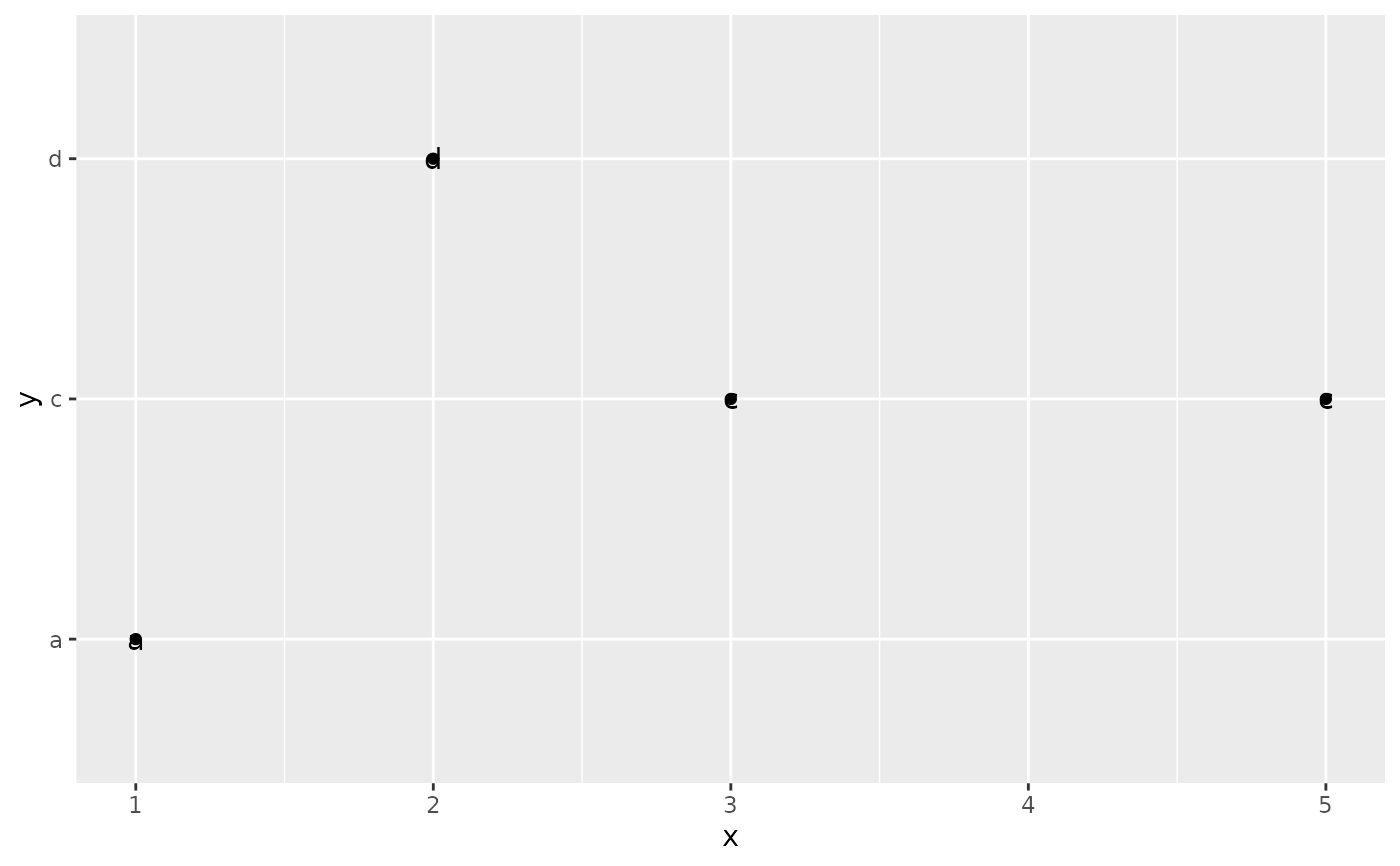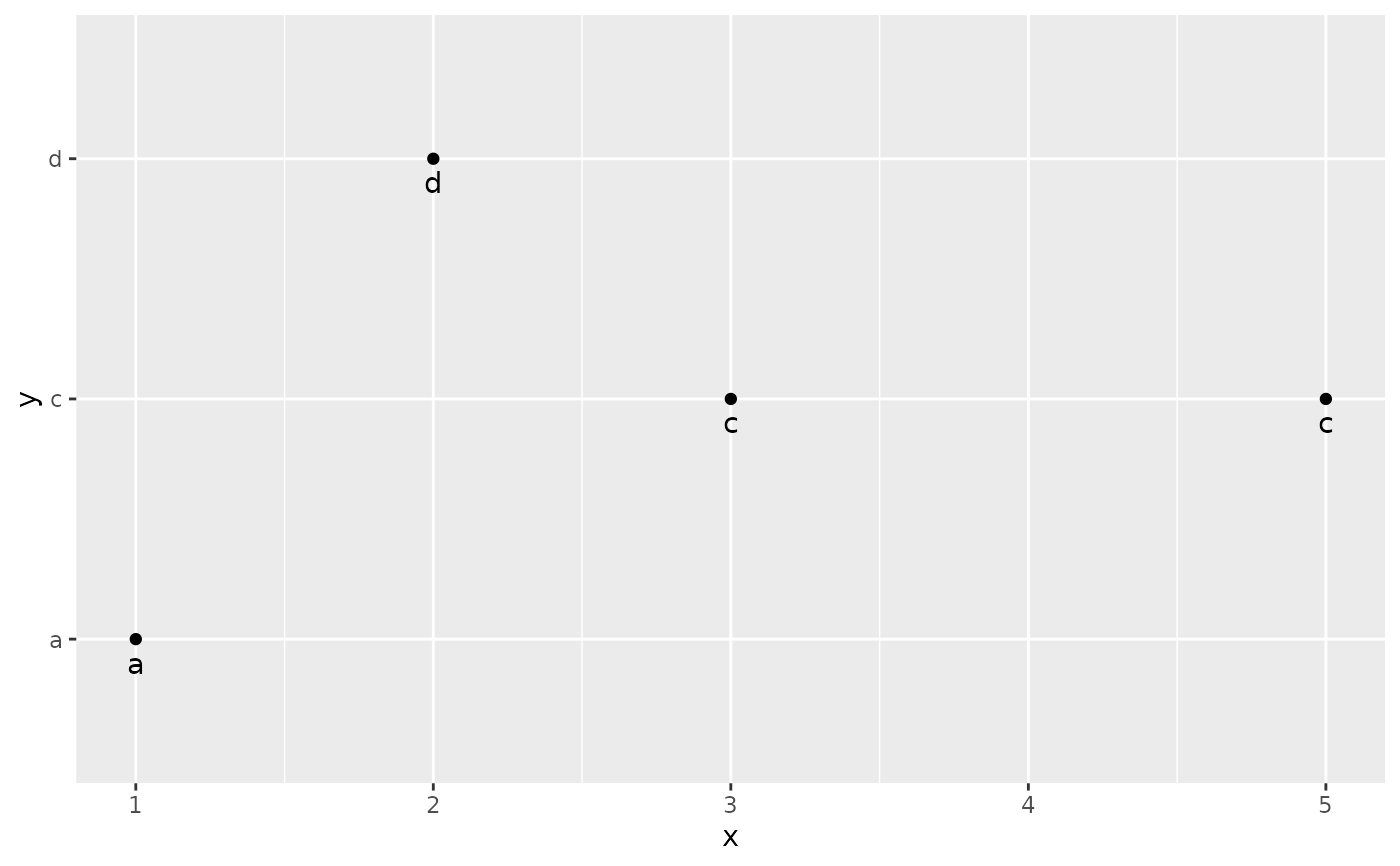position_nudge() is generally useful for adjusting the position of items on discrete scales by a small amount. Nudging is built in to geom_text() because it's so useful for moving labels a small distance from what they're labelling.

## Usage

position_nudge(x = 0, y = 0)

## Arguments

x, y

Amount of vertical and horizontal distance to move.

Other position adjustments: position_dodge(), position_identity(), position_jitterdodge(), position_jitter(), position_stack()

## Examples

df <- data.frame(
x = c(1,3,2,5),
y = c("a","c","d","c")
)

ggplot(df, aes(x, y)) +
geom_point() +
geom_text(aes(label = y))ggplot(df, aes(x, y)) +
geom_point() +
geom_text(aes(label = y), position = position_nudge(y = -0.1))# Or, in brief
ggplot(df, aes(x, y)) +
geom_point() +
geom_text(aes(label = y), nudge_y = -0.1)